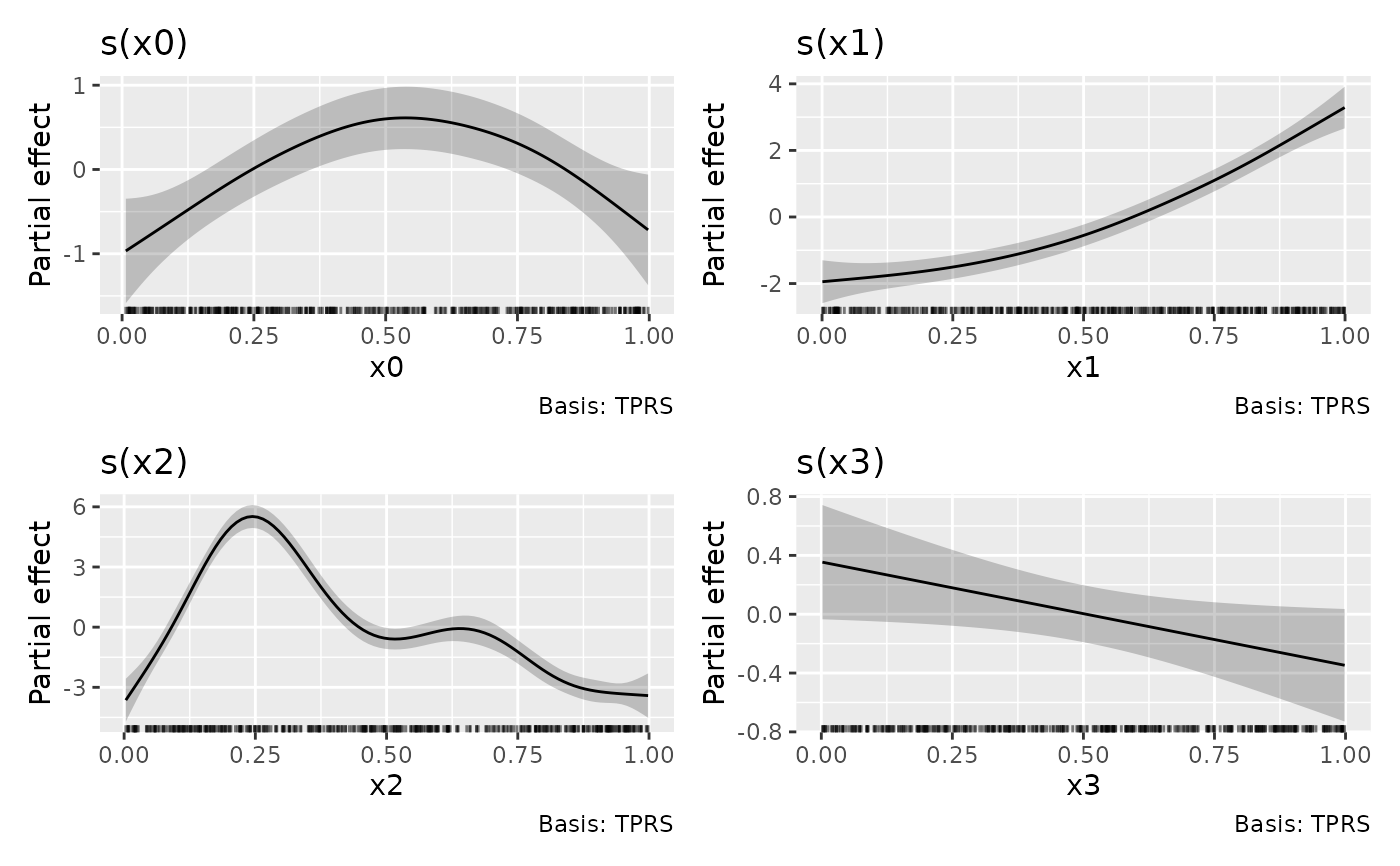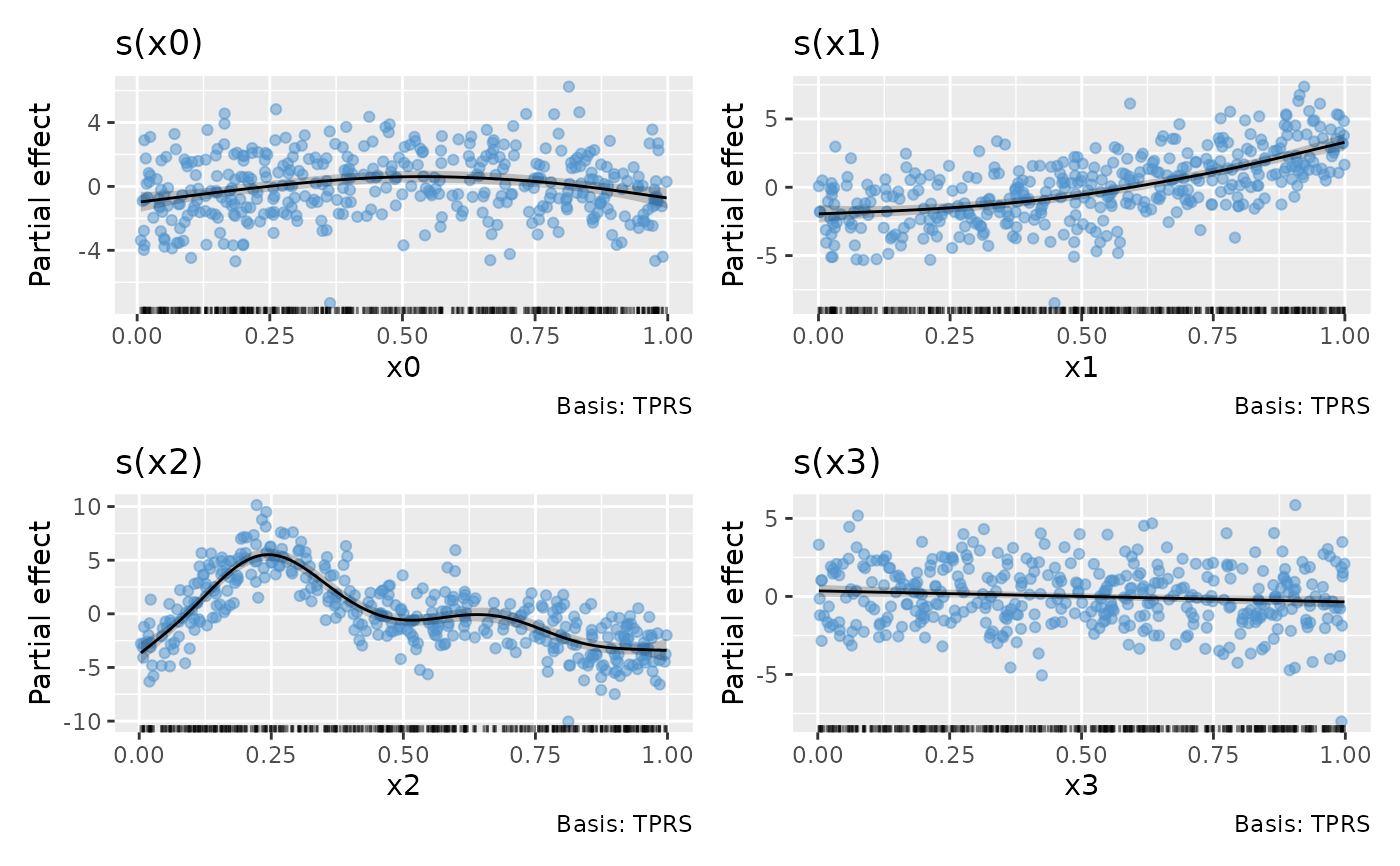Plots estimated smooths from a fitted GAM model in a similar way to mgcv::plot.gam() but instead of using base graphics, ggplot2::ggplot() is used instead.

## Usage

# S3 method for gam
draw(
object,
data = NULL,
select = NULL,
parametric = FALSE,
terms = NULL,
residuals = FALSE,
scales = c("free", "fixed"),
ci_level = 0.95,
n = 100,
n_3d = 16,
n_4d = 4,
unconditional = FALSE,
overall_uncertainty = TRUE,
constant = NULL,
fun = NULL,
dist = 0.1,
rug = TRUE,
contour = TRUE,
grouped_by = FALSE,
ci_alpha = 0.2,
ci_col = "black",
smooth_col = "black",
resid_col = "steelblue3",
contour_col = "black",
n_contour = NULL,
partial_match = FALSE,
discrete_colour = NULL,
discrete_fill = NULL,
continuous_colour = NULL,
continuous_fill = NULL,
position = "identity",
angle = NULL,
ncol = NULL,
nrow = NULL,
guides = "keep",
widths = NULL,
heights = NULL,
projection = "orthographic",
orientation = NULL,
wrap = TRUE,
envir = environment(formula(object)),
...
)

## Arguments

object

a fitted GAM, the result of a call to mgcv::gam().

data

a optional data frame that may or may not be used? FIXME!

select

character, logical, or numeric; which smooths to plot. If NULL, the default, then all model smooths are drawn. Numeric select indexes the smooths in the order they are specified in the formula and stored in object. Character select matches the labels for smooths as shown for example in the output from summary(object). Logical select operates as per numeric select in the order that smooths are stored.

parametric

logical; plot parametric terms also? Note that select is used for selecting which smooths to plot. The terms argument is used to select which parametric effects are plotted. The default, as with mgcv::plot.gam(), is to not draw parametyric effects.

terms

character; which model parametric terms should be drawn? The Default of NULL will plot all parametric terms that can be drawn.

residuals

logical; should partial residuals for a smooth be drawn? Ignored for anything but a simple univariate smooth.

scales

character; should all univariate smooths be plotted with the same y-axis scale? If scales = "free", the default, each univariate smooth has its own y-axis scale. If scales = "fixed", a common y axis scale is used for all univariate smooths.

Currently does not affect the y-axis scale of plots of the parametric terms.

ci_level

numeric between 0 and 1; the coverage of credible interval.

n

numeric; the number of points over the range of the covariate at which to evaluate the smooth.

n_3d

numeric; the number of new observations to generate for the third dimension of a 3D smooth.

n_4d

numeric; the number of new observations to generate for the dimensions higher than 2 (!) of a kD smooth (k >= 4). For example, if the smooth is a 4D smooth, each of dimensions 3 and 4 will get n_4d new observations.

unconditional

logical; should confidence intervals include the uncertainty due to smoothness selection? If TRUE, the corrected Bayesian covariance matrix will be used.

overall_uncertainty

logical; should the uncertainty in the model constant term be included in the standard error of the evaluate values of the smooth?

constant

numeric; a constant to add to the estimated values of the smooth. constant, if supplied, will be added to the estimated value before the confidence band is computed.

fun

function; a function that will be applied to the estimated values and confidence interval before plotting. Can be a function or the name of a function. Function fun will be applied after adding any constant, if provided.

dist

numeric; if greater than 0, this is used to determine when a location is too far from data to be plotted when plotting 2-D smooths. The data are scaled into the unit square before deciding what to exclude, and dist is a distance within the unit square. See mgcv::exclude.too.far() for further details.

rug

logical; draw a rug plot at the botom of each plot for 1-D smooths or plot locations of data for higher dimensions.

contour

logical; should contours be draw on the plot using ggplot2::geom_contour().

grouped_by

logical; should factor by smooths be drawn as one panel per level of the factor (FALSE, the default), or should the individual smooths be combined into a single panel containing all levels (TRUE)?

ci_alpha

numeric; alpha transparency for confidence or simultaneous interval.

ci_col

colour specification for the confidence/credible intervals band. Affects the fill of the interval.

smooth_col

colour specification for the smooth line.

resid_col

colour specification for the partial residuals.

contour_col

colour specification for contour lines.

n_contour

numeric; the number of contour bins. Will result in n_contour - 1 contour lines being drawn. See ggplot2::geom_contour().

partial_match

logical; should smooths be selected by partial matches with select? If TRUE, select can only be a single string to match against.

discrete_colour, discrete_fill, continuous_colour, continuous_fill

suitable scales for the types of data.

position

Position adjustment, either as a string, or the result of a call to a position adjustment function.

angle

numeric; the angle at which the x axis tick labels are to be drawn passed to the angle argument of ggplot2::guide_axis().

ncol, nrow

numeric; the numbers of rows and columns over which to spread the plots

guides

character; one of "keep" (the default), "collect", or "auto". Passed to patchwork::plot_layout()

widths, heights

The relative widths and heights of each column and row in the grid. Will get repeated to match the dimensions of the grid. If there is more than 1 plot and widths = NULL, the value of widths will be set internally to widths = 1 to accomodate plots of smooths that use a fixed aspect ratio.

projection

character; projection to use, see ggplot2::coord_map() for details.

orientation

an optional vector c(latitude, longitude, rotation) which describes where the "North Pole" should be when computing the projection. The third value is a clockwise rotation (in degrees), which defaults to the midrange of the longitude coordinates in the data. The default values for orientation therefore are c(20, 0, mean(range(longitude)))) if this is not specified by the user. See links in ggplot2::coord_map() for more information.

wrap

logical; wrap plots as a patchwork? If FALSE, a list of ggplot objects is returned, 1 per term plotted.

envir

an environment to look up the data within.

...

additional arguments passed to patchwork::wrap_plots().

## Value

The object returned is created by patchwork::wrap_plots().

## Note

Internally, plots of each smooth are created using ggplot2::ggplot() and composed into a single plot using patchwork::wrap_plots(). As a result, it is not possible to use + to add to the plots in the way one might typically work with ggplot() plots. Instead, use the & operator; see the examples.

Gavin L. Simpson

## Examples

load_mgcv()

# simulate some data
df1 <- data_sim("eg1", n = 400, dist = "normal", scale = 2, seed = 2)
# fit GAM
m1 <- gam(y ~ s(x0) + s(x1) + s(x2) + s(x3), data = df1, method = "REML")

# plot all smooths
draw(m1)draw(m1, residuals = TRUE)df2 <- data_sim(2, n = 1000, dist = "normal", scale = 1, seed = 2)
m2 <- gam(y ~ s(x, z, k = 40), data = df2, method = "REML")
draw(m2, contour = FALSE, n = 50)# change the number of contours drawn and the fill scale used for
# the surface
library("ggplot2")
draw(m2, n_contour = 5, n = 50,
continuous_fill = scale_fill_distiller(palette = "Spectral",
type = "div"))# See https://gavinsimpson.github.io/gratia/articles/custom-plotting.html
# for more examples and for details on how to modify the theme of all the
# plots produced by draw(). To modify all panels, for example to change the
# theme, use the & operator
`